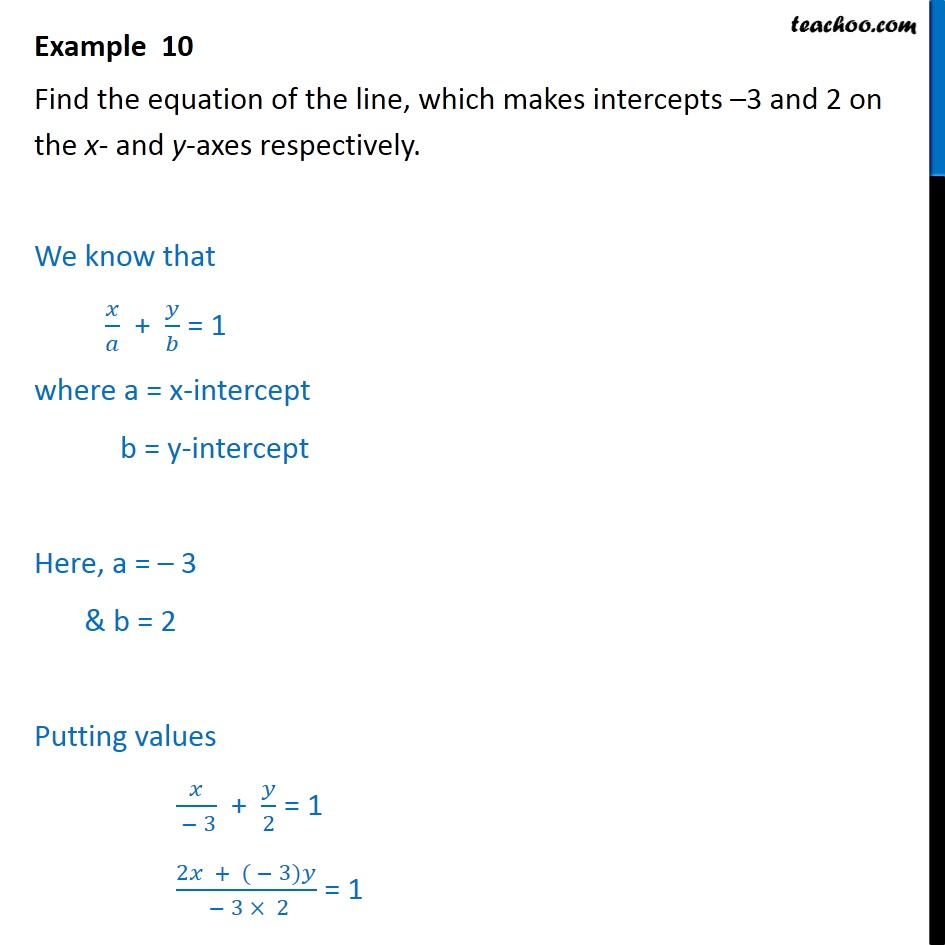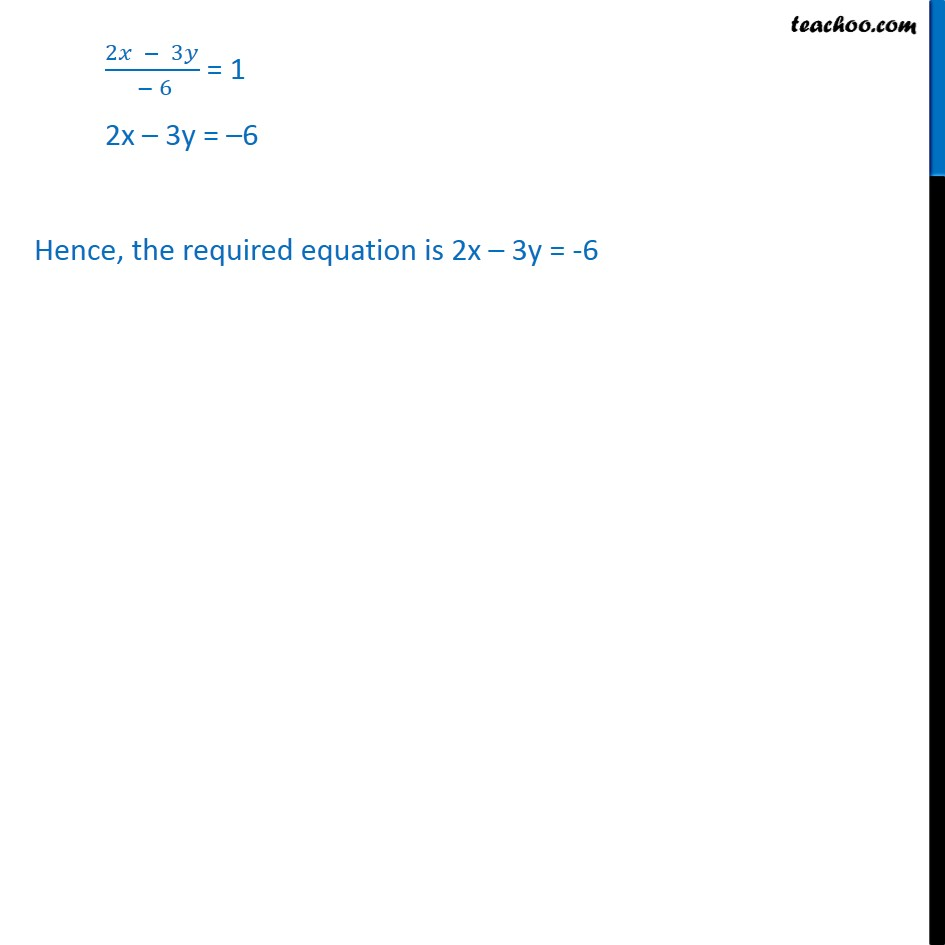Examples

Chapter 9 Class 11 Straight Lines
Serial order wiseLearn in your speed, with individual attention - Teachoo Maths 1-on-1 Class

### Transcript

Example 10 Find the equation of the line, which makes intercepts 3 and 2 on the x- and y-axes respectively. We know that / + / = 1 where a = x-intercept b = y-intercept Here, a = 3 & b = 2 Putting values /( 3) + /2 = 1 (2 + ( 3) )/( 3 2) = 1 (2 3 )/( 6) = 1 2x 3y = 6 Hence, the required equation is 2x 3y = -6